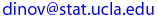## UCLA Statistics, Spring 2003

 Instructor: Ivo D. Dinov, Ph.D. Assistant Professor in Statistics, Research Scientist, Department of Neurology, UCLA School of Medicine E-mail:Teaching Assistan(s): Brian Ng  E-mail: bybn@stat.ucla.edu

Textbook:  Probability and Statistics for Engineering and the Sciences, Jay Devore, Duxbury, 5th edition (2000).
Tentative schedule of topics to be covered
1. Review:  Statistics Summaries, Population vs Sample, Plots, Probability, Independence, Bayes Theorem, Discrete & Continuous random variables: Binomial, Negative Binomial, Hypergeometric, Poisson, Normal , Exponential distribution, Joint vs. Marginal distribution, Central Limit Theorem, Bias & Efficiency, Confidence Intervals.
2. Confidence intervals: CI for the variance of a normal population (Ch. 01-06)
3. Hypothesis testing on a single sample: One-sided and two-sided tests, Type I and II errors (Ch. 07)
4. Hypothesis testing on a single sample: p-values (Ch. 08)
5. Inferences on two samples: Confidence intervals and Hypothesis testing for a difference between means
6. Inferences on two samples: proportions, paired data (Ch. 09)
7. Inferences on two samples: equal variances
8. The Analysis of Variance: Comparison of means of more than two populations (Ch. 10)
9. The Analysis of Variances: Unequal sample size (Ch. 11)
10. Simple Linear Regression: Scatterplot, Least squares estimates, interpretations, Confidence intervals (Ch. 12)
11. Simple Linear Regression: Prediction intervals, Diagnostics, Transformations
12. Multiple regression: Least square estimates, CI and prediction intervals, Dummy variables (Ch. 13)
13. Chi-Square (χ2) Goodness of Fit Test (Ch. 14)

Last modified on by.

Ivo D. Dinov, Ph.D., Departments of Statistics and Neurology, UCLA School of Medicine